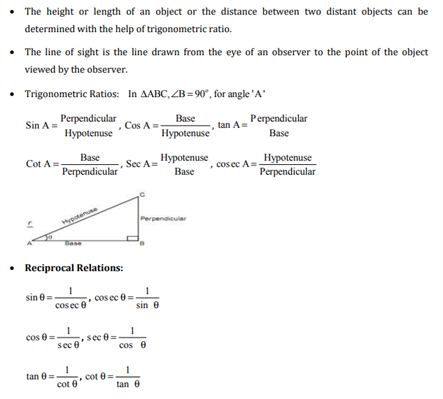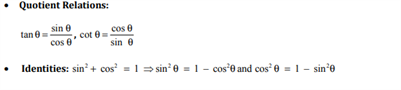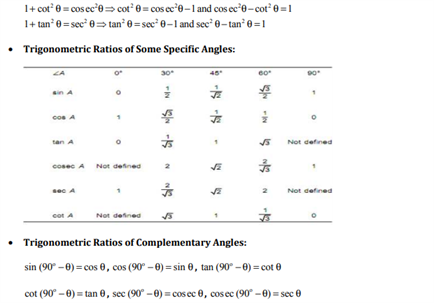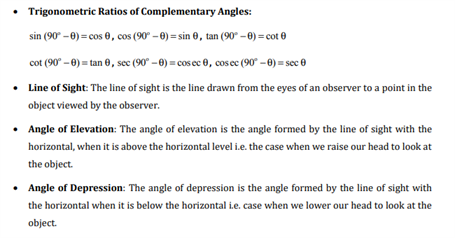# Revision Notes For CBSE Class 10 Maths Chapter 9 Some Applications of Trigonometry

Studying Chapter 9 is actually fun because this is where students learn about some of the ways in which trigonometry is used practically in our day to day lives. The chapter talks about the application of trigonometry in different fields like astronomy, geography and navigation. However, the main focus of this chapter is to help students learn about finding the heights and distances of various objects using trigonometry. As students will need to go through some important concepts like the angle of elevation, the angle of depression, and line of sight, etc. CBSE revision notes for class 10 maths chapter 9 – applications of trigonometry is provided here to help them clear all their doubts regarding the topics mentioned in this chapter. These notes further contain well-defined information that will aid students in easily mastering chapter concepts and studying efficiently. These notes can be downloaded freely.

Class 10 maths chapter 9 CBSE revision notes PDF can be viewed below.Access CBSE Class 10 Maths Sample Papers Here.

Access NCERT Class 10 Maths Book Here.

Also Read;

 NCERT Related Articles NCERT Solutions for Class 12 NCERT Solutions for Class 11 NCERT Solutions for Class 10 NCERT Solutions for Class 12 Maths NCERT Solutions for Class 11 Maths NCERT Solutions for Class 10 Maths NCERT Solutions for Class 12 Physics NCERT Solutions for Class 11 Physics NCERT Solutions for Class 10 Science NCERT Solutions for Class 12 Chemistry NCERT Solutions for Class 11 Chemistry NCERT Solutions for Class 6 NCERT Solutions for Class 12 Biology NCERT Solutions for Class 11 Biology NCERT Solutions for Class 6 Maths NCERT Solutions for Class 9 NCERT Solutions for Class 8 NCERT Solutions for Class 6 Science NCERT Solutions for Class 9 Maths NCERT Solutions for Class 8 Maths NCERT Solutions for Class 4 NCERT Solutions for Class 9 Science NCERT Solutions for Class 8 Science NCERT Solutions for Class 4 Maths NCERT Solutions for Class 5 NCERT Solutions for Class 7 NCERT Solutions for Class 7 Maths NCERT Solutions for Class 4 Science NCERT Solutions for Class 5 Maths NCERT Solutions for Class 7 Science NCERT Solutions NCERT Books NCERT Solutions for Class 5 Science